# Division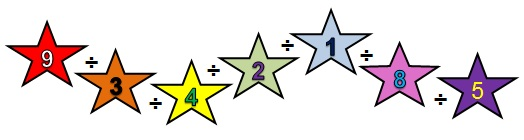It helps to know your times tables! Click on the times tables picture.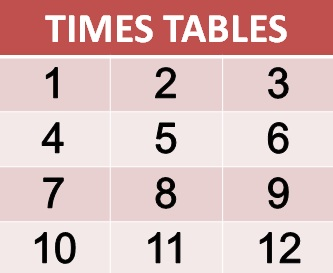## Dividing by 10

A decimal point comes after a whole number e.g. 2.1 - the number two is a whole number.

Move the decimal point one place to the left.

2 ÷ 10 = ?

Before
ThHTU1/10
2
After
ThHTU1/10
2

30 ÷ 10 = ?

Before
ThHTU1/10
30
After
ThHTU1/10
30

## Dividing by 100

Move the decimal point two places to the left.

750 ÷ 100 = ?

Before
ThHTU1/101/100
750
After
ThHTU1/101/100
750

## Dividing by 1000

Move the decimal point three places to the left.

4000 ÷ 1000 = ?

Before
ThHTU1/101/1001/1000
4000

After
ThHTU1/101/1001/1000
4000

# Dividing Numbers

If you know your times tables the following divisions will be easy for you to calculate:

## Example 1

42 ÷ 7 = ?

We know 6 x 7 = 42.

Therefore 42 ÷ 7 = 6.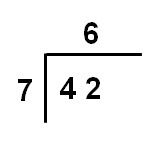## Example 2

32 ÷ 8 = ?

We know 4 x 8 = 32.

Therefore 32 ÷ 8 = 4.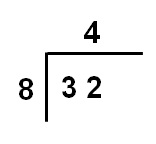## Example 3

25 ÷ 5 = ?

We know 5 x 5 = 25.

Therefore 25 ÷ 5 = 5.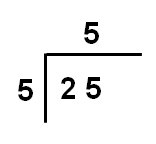# Graphical Items

Let's have a look at graphical items.

## Example 1

If you have nine balls and three people. How many balls would
each person have?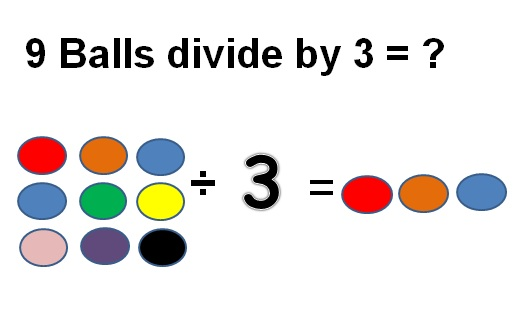## Example 2

If you have 4 presents and two people. How many presents would each person have?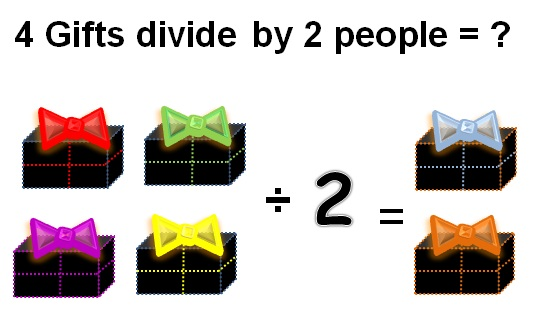# Division with Remainders

In division you have one or more remainders left.

## Example 1

42 ÷ 3 = ?

1. 4 ÷ 3 = 1 plus 1 remainder
2. Carry the remainder 1.
3. 12 ÷ 3 = 4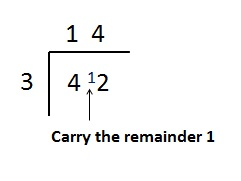## Example 2

95 ÷ 5= ?

1. 9 ÷ 5 = 1 plus 4 remainders
2. Carry the 4 remainders.
3. 45 ÷ 5 = 9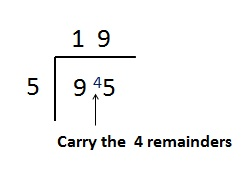## Example 3

66 ÷ 12 = ?

1. You know 12 x 5= 60 so 66 ÷ 12 = 5 plus 6 remainders.
2. Add a decimal point after the 5.
3. After the 6 remainders add a 0.
4. 60 ÷ 12 = 5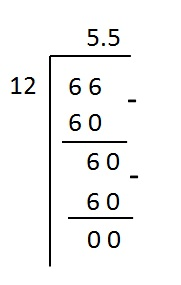# VIDEO

Have a look at the demonstration on the video to enhance your learning.Now try the Exercises!